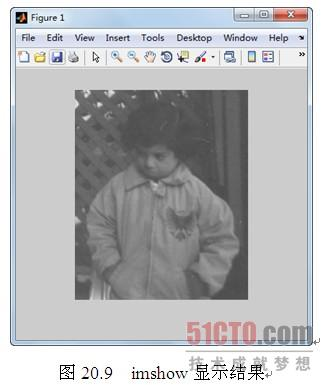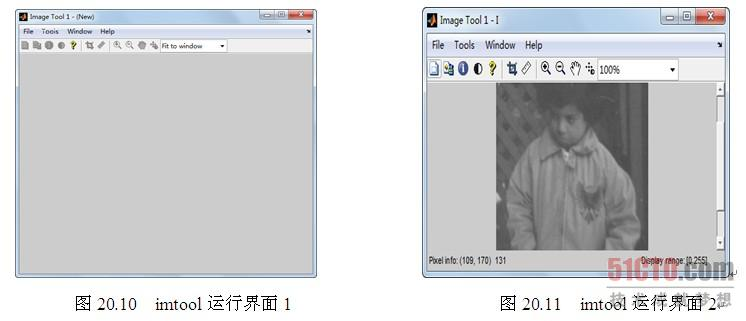• matlab开发-调整数组和图像的大小。y=调整大小（x，newSize）使用离散余弦变换调整输入数组x的大小。
• Matlab读取16进制RGB文档转化为彩色图像，包括matlab程序，材料RGB三色文档，和是一幅1080*1920的空图像。和操作说明
• MATLAB元胞数组（cell）可以将浮点型、字符型、结构数组等不同类型的数据放在同一个存储单元中 [c,1]cell中插入数字1 cell2mat(c(2)) — cell类型转换为矩阵 读取图片 %% 读取图片 clc, clear, close all a1=...

第三章

元胞数组

1. MATLAB元胞数组（cell）可以将浮点型、字符型、结构数组等不同类型的数据放在同一个存储单元中
2. [c,1]cell中插入数字1 cell2mat(c(2)) — cell类型转换为矩阵

读取图片

%% 读取图片
clc, clear, close all
[m,n]=size(a1);
%% 批量读取图片
dirname = 'ImageChips';
files = dir(fullfile(dirname, '*.bmp'));
a=zeros(m,n,19);
pic=[];
for ii = 1:length(files)
filename = fullfile(dirname, files(ii).name);
pic=[pic,a(:,:,ii)];
end
double(pic);
figure
imshow(pic,[])

1. dir(xxx)返回其中文件名或所有文件夹下所有文件名
2. fullfile(a,b,c)合成一个地址a\b\c
3. a(:,:,1)分块的矩阵（其中的第一块）
5. 原理在于将图片变为矩阵，通过拼接ju’z矩阵来转换为图片。

批量行拼接图片

function ConnectImages()
%% 读取图片
clc, clear, close all

%% 批量拼接图片

files = dir('*.jpg');maxrows=0;maxlines=0;
pic=[];rows=[];lines=[];
for ii = 1:length(files)
filename = fullfile(files(ii).name);
[m,n]=size(lite);
rows=[rows m];lines=[lines n];
end
maxrows=max(rows);

for ii = 1:length(files)
filename = fullfile(files(ii).name);
[m,n]=size(lite2);
temp=[lite2 ];
final=[temp;255.*ones(maxrows-m,n/3,3)];
pic=[pic,final];
end

figure
imshow(pic,[])
end

批量行加密图片

files = dir('*.jpg');
for ii = 1:length(files)
filename = fullfile(files(ii).name);
[m,n]=size(lite);
encry=zeros(m,n/3,3);
key=randsample(m,m)';
for k=1;3
encry(:,:,k)=lite(key,:,k);
end
encry=uint8(encry);
figure
imshow(encry);
end

杂项

• 右键表格文件（xls）直接导入数据，选择列向量可以直接看到、选用内部子列
• 如果选择导出脚本会看到导入的具体代码，并加以修改、发布
• maxdrawdown()计算最大回撤
• 三维数组中每一个size都要相等
展开全文MATLAB
• 20.1.4 显示图像数据MATLAB图像处理工具箱有两个函数，imshow和imtools可以用于数字图像的显示。imshow是基本的显示函数，例如，对前面已经读入的图像数据I(pout图像数据)，在MATLAB命令窗口输入以下代码。>>...

20.1.4  显示图像数据

MATLAB图像处理工具箱有两个函数，imshow和imtools可以用于数字图像的显示。imshow是基本的显示函数，例如，对前面已经读入的图像数据I(pout图像数据)，在MATLAB命令窗口输入以下代码。

>>imshow(I)

运行结果如图20.9所示。对于索引图的显示，用户需要传递一个Colormap给imshow，否则按灰度图显示。例如，在MATLAB命令窗口输入以下代码。

>>imshow(X,map);

imtool是MATLAB图像处理工具箱的一个交互式图像处理工具，除了显示图像，还能完成很多图像处理的任务。在MATLAB命令窗口输入以下代码。

>>imtool

运行上述代码得到imtool的运行界面如图20.10所示。根据图20.10中光标的提示，导入工作区间中的图形数据I，得到imtool图像处理界面，如图20.11所示。一种更有效的方式是直接输入以下指令，得到图20.11所示的界面。通过这个工具无须输入任何代码就可以完成一些比较复杂的图像处理任务。

>>imtool(I);函数montage可以同时显示多帧图像，其用法与imshow类似，对灰度图或二值图，其调用格式为：

montage(I)

对索引图，其调用格式为：

montage(I, map)

下面是一个简单的多帧图像显示示例。在MATLAB命令窗口输入以下代码。

mri=uint8(zeros(128,128,1,27));

forframe=1:27

end

montage(mri,map);

上述代码运行结果如图20.12所示。【责任编辑：book TEL：(010)68476606】

点赞 0

展开全文• 这里写自定义目录标题欢迎使用Markdown编辑器新的改变功能快捷键合理的创建标题，有助于目录的生成如何改变文本的样式插入链接与图片如何插入一段漂亮的代码片生成一个适合你的列表创建一个表格设定内容居中、居左、...

语法：
pks = findpeaks(data)
[pks,locs] = findpeaks(data)
[pks,locs,w,p] = findpeaks(data)
[___] = findpeaks(data,x)
[___] = findpeaks(data,Fs)
[___] = findpeaks(___,Name,Value)

展开全文matlab
• 以下内容来自于：...4. 3.1 结构数组的创建MATLAB提供了两种定义结构的方式：直接法和使用struct函数法。1. 使用直接引用方式定义结构与建立数值型数组一样，...

以下内容来自于：https://blog.csdn.net/u010999396/article/details/54413615/

要在MALTAB中实现比较复杂的编程，就不能不用struct类型。而且在MATLAB中实现struct比C中更为方便。

4. 3.1 结构数组的创建

MATLAB提供了两种定义结构的方式：直接法和使用struct函数法。

1. 使用直接引用方式定义结构

与建立数值型数组一样，建立新struct对象不需要事先申明，可以直接引用，而且可以动态扩充。比如建立一个复数变量x：

x.real = 0; % 创建字段名为real，并为该字段赋值为0

x.imag = 0 % 为x创建一个新的字段imag，并为该字段赋值为0

x =

real: 0

imag: 0

然后可以将旗动态扩充为数组：

x(2).real = 0; % 将x扩充为1×2的结构数组

x(2).imag = 0;

在任何需要的时候，也可以为数组动态扩充字段，如增加字段scale：

x(1).scale = 0;

这样，所有x都增加了一个scale字段，而x(1)之外的其他变量的scale字段为空：

x(1) % 查看结构数组的第一个元素的各个字段的内容

ans =

real: 0

imag: 0

scale: 0

x(2) % 查看结构数组的第二个元素的各个字段的内容，注意没有赋值的字段为空

ans =

real: 0

imag: 0

scale: []

*******************************************************************************************

2. 使用struct函数创建结构

使用struct函数也可以创建结构，该函数产生或吧其他形式的数据转换为结构数组。

struct的使用格式为：

s = sturct('field1',values1,'field2',values2,…);

该函数将生成一个具有指定字段名和相应数据的结构数组，其包含的数据values1、valuese2等必须为具有相同维数的数据，数据的存放位置域其他结构位置一一对应的。对于struct的赋值用到了元胞数组。数组values1、values2等可以是元胞数组、标量元胞单元或者单个数值。每个values的数据被赋值给相应的field字段。

当valuesx为元胞数组的时候，生成的结构数组的维数与元胞数组的维数相同。而在数据中不包含元胞的时候，得到的结构数组的维数是1×1的。例如：

s = struct('type',{'big','little'},'color',{'blue','red'},'x',{3,4})

s =

1x2 struct array with fields:

type

color

x

得到维数为1×2的结构数组s，包含了type、color和x共3个字段。这是因为在struct函数中{'big','little'}、{'blue','red'}和{3,4}都是1×2的元胞数组，可以看到两个数据成分分别为：

s(1,1)

ans =

type: 'big'

color: 'blue'

x: 3

s(1,2)

ans =

type: 'little'

color: 'red'

x: 4

相应的，如果将struct函数写成下面的形式：

s = struct('type',{'big';'little'},'color',{'blue';'red'},'x',{3;4})

s =

2x1 struct array with fields:

type

color

x

则会得到一个2×1的结构数组。

展开全文• 5.3 Cell数组的基本操作本小节结合示例对元胞数组的一些基本操作进行介绍。【例3-26】元胞数组的合并。>> C1 = {'Jan' 'Feb'; '10' '17'; uint16(2004) uint16(2001)};>> C2 = {'Mar' 'Apr' 'May'; '31'...
• 直奔主题，需要保存处理图像的文件名(string)数组。本文只用作自己的实验记录，侵删。搬运自matlab官网的代码：https://www.mathworks.com/matlabcentral/fileexchange/7601-cell2csv?s_tid=mwa_osa_a以下为MATLAB ...
• 关键字：Matlab数组、函数、计算、绘图正文1、MATLAB 产品1.1 MATLAB 产品描述全世界数超过百万的工程师和科学家们使用 MATLAB 来分析和设计改变世界的系统和产品。MATLAB 应用于汽车安全系统、宇宙飞船、健康...matlab复数信号
• matlab如何求出一个数组中最接近某个数的两五个数，带有测试图片，要求大于五个只需要按程序一次添加相应的代码！
• matlab字符串数组、元胞数组提取数字背景示例代码 背景 最近需要整理一个图片测试集，其中需要将图片与对应主观评价分对号。由于部分序列集中图片顺序混乱，因此需要将图片的序号提取出来，网上有单字符串提取数字...
• 2D 案例的图片形式： 如果 A = [mxn 数组]，则B = repToSize(A,x,y) 产生| AA .. A A2 | | AA .. A A2 | | .. .. .. .. .. | | AA .. A A2 | | A1 A1 .. A1 A3 | 其中 x >= m，y >= n，并且A1 = A(1:rem(x,m),:) A2...matlab
• 1. Matlab数组元素引用有三种方法： - 下标法(subscripts) - 索引法(index) - 布尔法(Boolean) - Markdown和扩展Markdown简洁的语法 - 代码块高亮 - 图片链接和图片上传 - LaTex数学公式 - UML...matlab
• 在做毕业设计，遇到一些问题，所以就把问题和解决方法记录下来。源代码：feat = FADE(n_image); %提取特征index=round(rand(1,N)*length(feat));... %合并所有图片特征值在matlab中，数组下标是从1开始的，我检查...
• 下面介绍MATLAB中如何建立数组，以及数组的常用操作等，包括数组的算术运算、关系运算和逻辑运算，以及数组信息的获取等。 在MATLAB中，一般使用方括号([])、逗号(,)、空格及分号(;)来创建数组数组中同一行的元.....
• 1、uint8与doubledouble函数只是将读入图像的uint8数据转换为double类型，一般不使用；...MATLAB中读入图像的数据类型是uint8，而在矩阵中使用的数据类型是double。因此I2=im2double(I1) :把图像数组I1...
• 由于学校课程的需要，我们要学习Matlab来完成数字信号处理的实验，我会在每节课结束以后把所学Matlab的相关知识整理发布。 数组的生成 数组的生成 1.冒号法 %%生成一个长度为100，从0到99的数组 %%使用：(初值:步长:...matlab
• 元胞数组MATLAB的一种特殊数据类型，可以将元胞数组看做一种无所不包的通用矩阵，或者叫做广义矩阵。组成元胞数组的元素可以是任何一种数据类型的常数或者常量，每一个元素也可以具有不同的尺寸和内存占用空间，...
• matlab plot基本用法

千次阅读 2021-04-18 08:21:53
plot(y)生成的图形是以序号为横坐标、数组y的数值为纵坐标画出的折线。//////////////////////////////////////////////////////////////////////////////////////////////////>> x=linsp...
• 今天在看数字图像处理教材的时候，有提到人眼只能分辨40个灰度级，所以想着用MATLAB打印一幅灰度范围[0,255]渐变的图像。最开始的代码如下： clear ; clc; %生成768*768灰度矩阵 A=zeros(1,768); vector=ones(1,...MATLAB 图像处理php python 数据挖掘 自动驾驶
• MATLAB中外部数据读取并写入元胞数组的方法与步骤 由于最近做时序分类问题用到了LSTM，则不得不接触一下元胞数组这个概念了… 引用 matlab官方文档（textscan用法）：...matlab 元胞数组
• 代码m=255*335;n=33;A=zeros(m,n); // 创建一个空的二维数组for x=1:1:255 // x从1遍历到255，步长为1(是叫做步长吧)for y=1:1:335double i; //若是定义成int型的，因为85425超过了int型的最...
• python、matlab高维数组转置

千次阅读 2018-12-17 14:38:19
转置可以对数组进行重置，返回的是源数据的视图（不会进行任何复制操作）。 转置有三种方式，transpose方法、T属性以及swapaxes方法。 1 .T,适用于一、二维数组 In : import numpy as np In : arr = np.a...
• matlab 报错 索引超出数组元素的数目(1)。

万次阅读 多人点赞 2021-03-29 14:44:58
matlab 报错 索引超出数组元素的数目(1)。 ** 1：首先我们要明白这个报错的含义。索引超出数组元素的数目**（1）。**要明白这个（1）的意思其实就是 “你当前引用的数组元素的个数超过了这个数组定义的个数“。其实...matlab...

matlab图片数组matlab 订阅